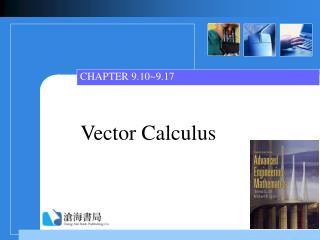DownloadDownload PresentationVector Calculus

# Vector Calculus

Télécharger la présentation## Vector Calculus

- - - - - - - - - - - - - - - - - - - - - - - - - - - E N D - - - - - - - - - - - - - - - - - - - - - - - - - - -
##### Presentation Transcript

1. CHAPTER 9.10~9.17 Vector Calculus

2. Contents • 9.10 Double Integrals • 9.11 Double Integrals in Polar Coordinates • 9.12 Green’s Theorem • 9.13 Surface Integrals • 9.14 Stokes’ Theorem • 9.15 Triple Integrals • 9.16 Divergence Theorem • 9.17 Change of Variables in Multiple Integrals

3. 9.10 Double Integrals • Recall from Calculus • Region of Type ISee the region in Fig 9.71(a) R: a x  b, g1(y)  y  g2(y) • Region of Type IISee the region in Fig 9.71(b) R: c  y  d, h1(x)  x  h2(x)

4. Fig 9.71

5. Iterated Integral • For Type I: (4) • For Type II: (5)

6. THEOREM 9.12 Let f be continuous on a region R. (i) For Type I: (6)(ii) For Type II: (7) Evaluation of Double Integrals

7. Note: • Volume =wherez = f(x, y) is the surface.

8. Example 1 Evaluate over the region bounded by y = 1, y = 2, y = x, y = −x + 5.See Fig 9.73. SolutionThe region is Type II

9. Fig 9.73

10. Example 2 Evaluate over the region in the first quadrant bounded by y = x2, x = 0, y = 4. SolutionFrom Fig 9.75(a) , it is of Type IHowever, this integral can not be computed.

11. Fig 9.75(a) Fig 9.75(b)

12. Example 2 (2) Trying Fig 9.75(b), it is of Type II

13. Method to Compute Center of Mass • The coordinates of the center of mass are (10)where (11)are the moments. Besides, (x, y) is a variable density function.

14. Example 3 A lamina has shape as the region in the first quadrant that is bounded by the y= sin x, y = cos x between x = 0and x = 4.Find the center of mass if (x, y) = y. SolutionSee Fig 9.76.

15. Example 3 (2)

16. Example 3 (3)

17. Example 3 (4)

18. Example 3 (5) Hence

19. Moments of Inertia • (12)are the moments of inertia about the x-axis and y-axis, respectively.

20. Example 4 Refer to Fig 9.77. Find Iy of the thin homogeneous disk of mass m. Fig 9.77

21. Example 4 (2) Solution Since it is homogeneous, the density is the constant (x, y) = m/r2.

22. Example 4 (3)

23. Radius of Gyration • Defined by (13)In Example 4,

24. 9.11 Double Integrals in Polar Coordinates • Double IntegralRefer to the figure.The double integral is

25. Refer to the figure.The double integral is

26. Example 1 Refer to Fig 9.83. Find the center of mass wherer = 2 sin 2 in the first quadrant and  is proportional to the distance from the pole. Fig 9.83

27. Example 1 (2) Solution We have: 0    /2,  = kr, then

28. Example 1 (3) Since x = r cos andthen

29. Example 1 (4) Similarly, y = r sin  , then

30. Change of Variables • Sometimes we would like to change the rectangular coordinates to polar coordinates for simplifying the question. If and then (3)Recall: x2 + y2 = r2 and

31. Example 2 Evaluate SolutionFrom the graph is shown in Fig 9.84.Using x2 + y2 = r2, then 1/(5 + x2 + y2 ) = 1/(5 + r2)

32. Fig 9.84

33. Example 2 (2) Thus the integral becomes

34. Example 3 Find the volume of the solid that is under and above the region bounded byx2 + y2 – y = 0. See Fig 9.85. SolutionFig 9.85

35. Example 3 (2) We find that and the equations becomeand r = sin . Now

36. Example 3 (3)

37. Area • If f(r, ) = 1,then the area is

38. 9.12 Green’s Theorem • Along Simply Closed Curves For different orientations for simply closed curves, please refer to Fig 9.88.Fig 9.88(a) Fig 9.88(b) Fig 9.88(c)

39. Notations for Integrals Along Simply Closed Curves • We usually write them as the following formswhere and represents in the positive and negative directions, respectively.

40. THEOREM 9.13 • Partial ProofFor a region R is simultaneously of Type I and Type II, IF P, Q, P/y, Q/x are continuous on R, which is bounded by a simply closed curve C, then Green’s Theorem in the Plane

41. Fig 9.89(a) Fig 9.89(b)

42. Partial Proof Using Fig 9.89(a), we have

43. Partial Proof Similarly, from Fig 9.89(b),From (2) + (3), we get (1).

44. Note: • If the curves are more complicated such as Fig 9.90, we can still decompose R into a finite number of subregions which we can deal with. • Fig 9.90

45. Example 1 • Evaluate where C is shown in Fig 9.91.

46. Example 1 (2) SolutionIf P(x, y) = x2 – y2, Q(x, y) = 2y – x, thenandThus

47. Example 2 Evaluate where C is the circle (x – 1)2 + (y – 5)2 = 4 shown in Fig 9.92.

48. Example 2 (2) SolutionWe have P(x, y) = x5 + 3yandthenHence Since the area of this circle is 4, we have

49. Example 3 • Find the work done by F = (– 16y + sin x2)i + (4ey + 3x2)j along C shown in Fig 9.93.

50. Example 3 (2) SolutionWe have Hence from Green’s theorem In view of R, it is better handled in polar coordinates, since R: